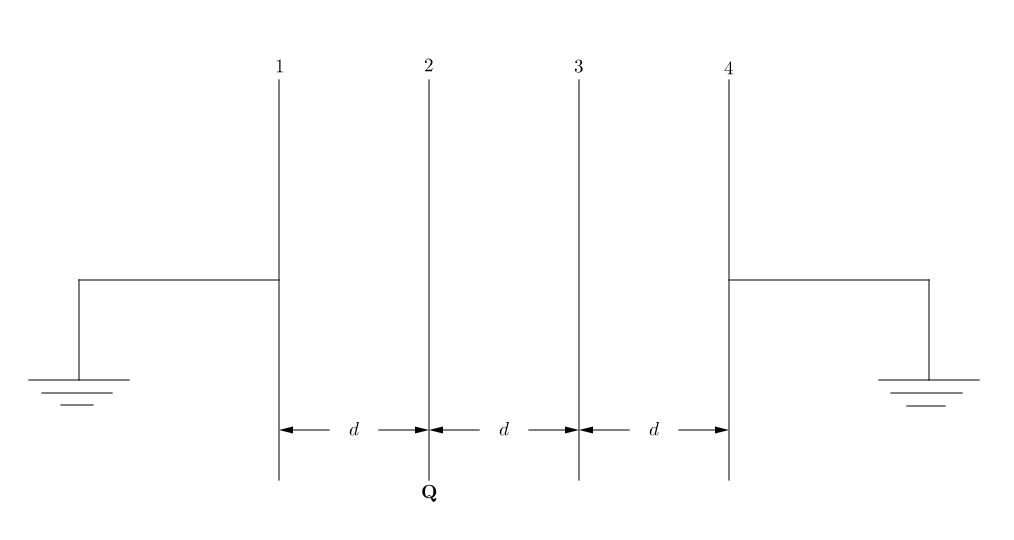# Charged plates, one after the other.Four identical metallic plates (initially uncharged), each having area of cross section $A,$ are each separated by a distance of $d,$ as shown in the figure above. Plate 2 is given a charge of $\mathbf Q.$ Plates 1 and 4 are both earthed via a very thin conducting wire. Find the potential difference between plates 2 and 3.


Details and Assumptions:

• $\epsilon_0$ denotes the permittivity of free space.
• The plates are very large, and the phenomena of fringing of electric field lines are neglected.
• Because of the large size, the electric field due to any particular plate is considered as that of an infinitely large, thin conducting plate.

Try a harder version.

×